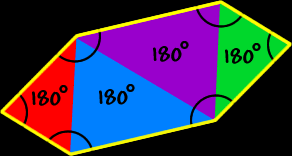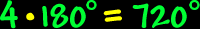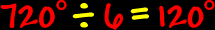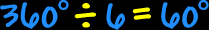# Polygons - Hexagons

Properties of hexagons, interior angles of hexagons

Polygons:  Properties of HexagonsSum of the Interior Angles of a Hexagon:To find the sum of the interior angles of a hexagon, divide it up into triangles... There are four triangles...  Because the sum of the angles of each triangle is 180 degrees...  We getSo, the sum of the interior angles of a hexagon is 720 degrees.Regular Hexagons:
The properties of regular hexagons:All sides are the same length (congruent) and all interior angles are the same size (congruent).

To find the measure of the interior angles, we know that the sum of all the angles is 720 degrees (from above)...  And there are six angles...So, the measure of the interior angle of a regular hexagon is 120 degrees.The measure of the central angles of a regular hexagon:To find the measure of the central angle of a regular hexagon, make a circle in the middle...  A circle is 360 degrees around...  Divide that by six angles...So, the measure of the central angle of a regular hexagon is 60 degrees.A regular hexagon is made up of 6 equilateral triangles!